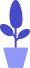# Python practical training project

Estimated reading: 2 minutes 90 views

Through the previous study, we already know how to use variables, numbers and strings in Python.

Next, we combine the knowledge introduced above to create a report card to record the grades of the students in the class.

• First, we need to use the input() function to prompt the user for a student ID and name.
• Then, the user is required to enter Chinese grades, math grades, and English grades.
• Next, we will use the print() function to print the student’s student number, name, Chinese grade, math grade, English grade, and the total score of the three homework.

Because the input scores of each subject are of string type, in order to calculate the total score, we also need to use the int() function to convert the string type to a number type for sum calculation. The code looks like this:

``````name = input("Please enter the student's name:")
userID = input("Please enter the student's student ID:")
score1 = input("Student's Engle language score:")
score2 = input("Student's math score:" )
score3 = input("Student's English score:")
total = float(score1) + float(score2) + float(score3)
print ("--------------")
print ("Student ID Name Chinese Math English Total Score")
print (userID," " , name, " ",score1, " ",score2, " ",score3," ",total)
``````

We save this code to the D:\Python Programs\ch02 directory and name the program file 2.1.py.

After running the program, its output is shown in Figure 1.